Home > CAAC > Chapter 7 > Lesson 7.3.4 > Problem7-112

7-112.
1. Complete each generic rectangle below and write the area as a sum and as a product. Homework Help ✎

1.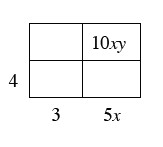2.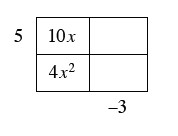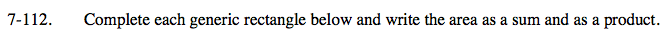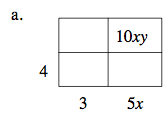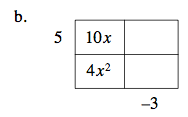First figure all of the dimensions of the rectangle by dividingthe known area by the given side.

10xy divided by 5x is 2y.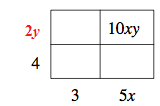To show the area as a product, write the dimensions multiplied together.

(2y + 4)(5x + 3)

Find the areas of all of the sections by multiplying the dimensions together, then add the areas to express as a sum.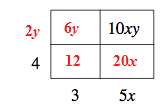6y + 20x + 10xy + 12

Use the same method from part (a) to solve part (b).